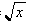MAT103
Franklin College
Erich Prisner

# The inverse function of a composed function

The inverse function of f(x) = x+1 is f-1(x) = x-1. The inverse function of g(x) =is g-1(x) = x2. Can the inverse of the composed function g(f(x)) =be expressed by the inverses of f and g? Is this true in general? Discuss also whether or not a composed function of two 1-1 functions must be 1-1. Discuss also special cases: Is the composed function of two (strictly) increasing/decreasing functions again (strictly) increasing/decreasing?

Erich Prisner, August 2003